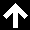# \$100,000 per Bitcoin

By Yaroslav Krasko Updated November 21, 2021

We recently posted an article about one of the most famous Stock to Flow model on BTC and its founder Plan B, who claims that bitcoin at the end of this year will cost 135 thousand dollars. Nobody can tell whether bitcoin will cost 135 thousand dollars, we will check it only with time.

KEY ISSUES:

• how much can bitcoin rise?
• how much will bitcoin rise in 2021?
• what would bitcoin rise to?

In this article, we will look at three more potential models that can anticipate and help us find the maximum and sell bitcoin at the best prices.

## The first model is the average growth rate of bitcoin

For this method, we chose the BLX index, which shows the entire history of bitcoin and the monthly time frame.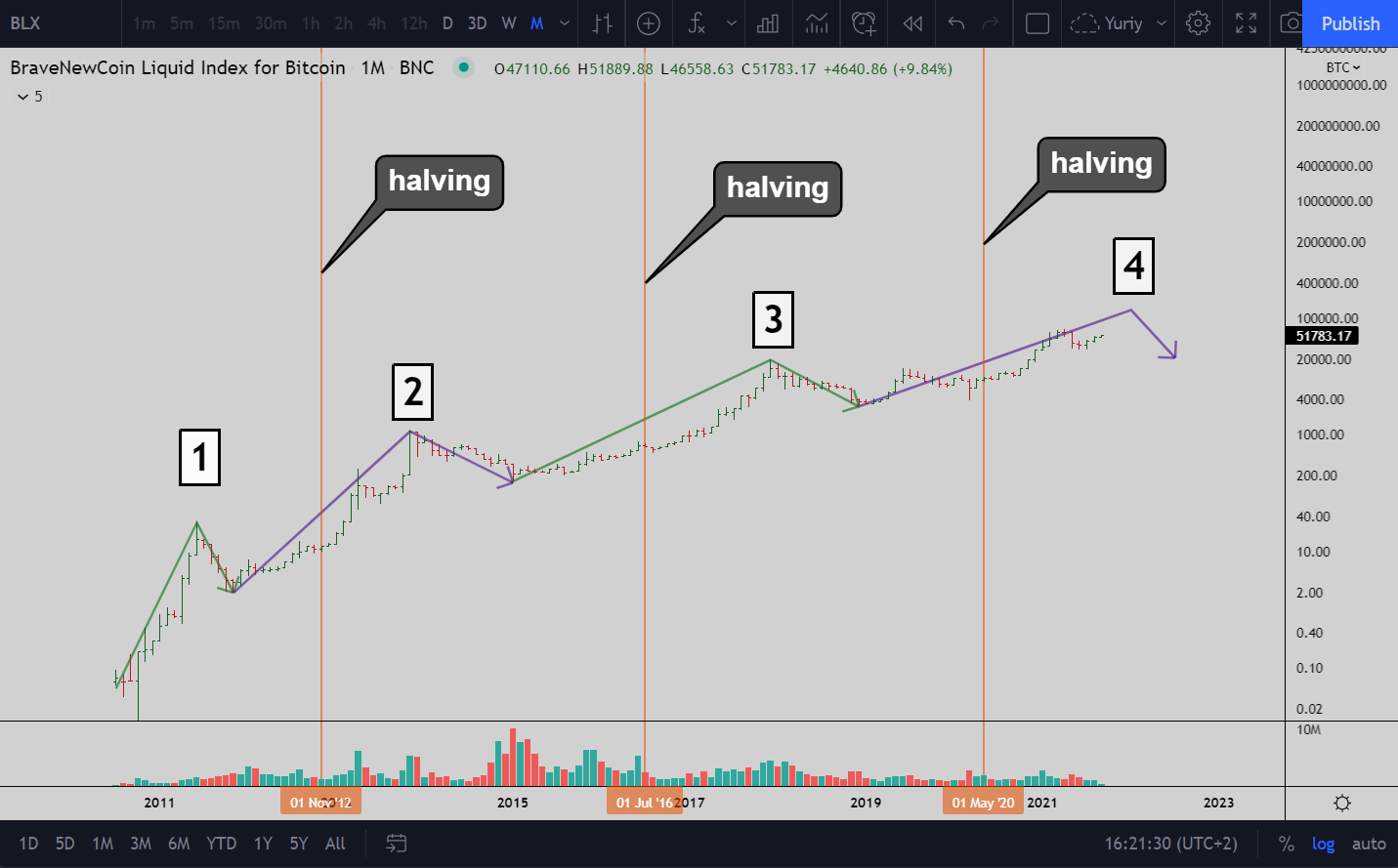The chart can be divided into so-called bitcoin cycles. Yeah, this data may not be enough to collect good statistics and build a trading system based on this data, but we have to work with what we have.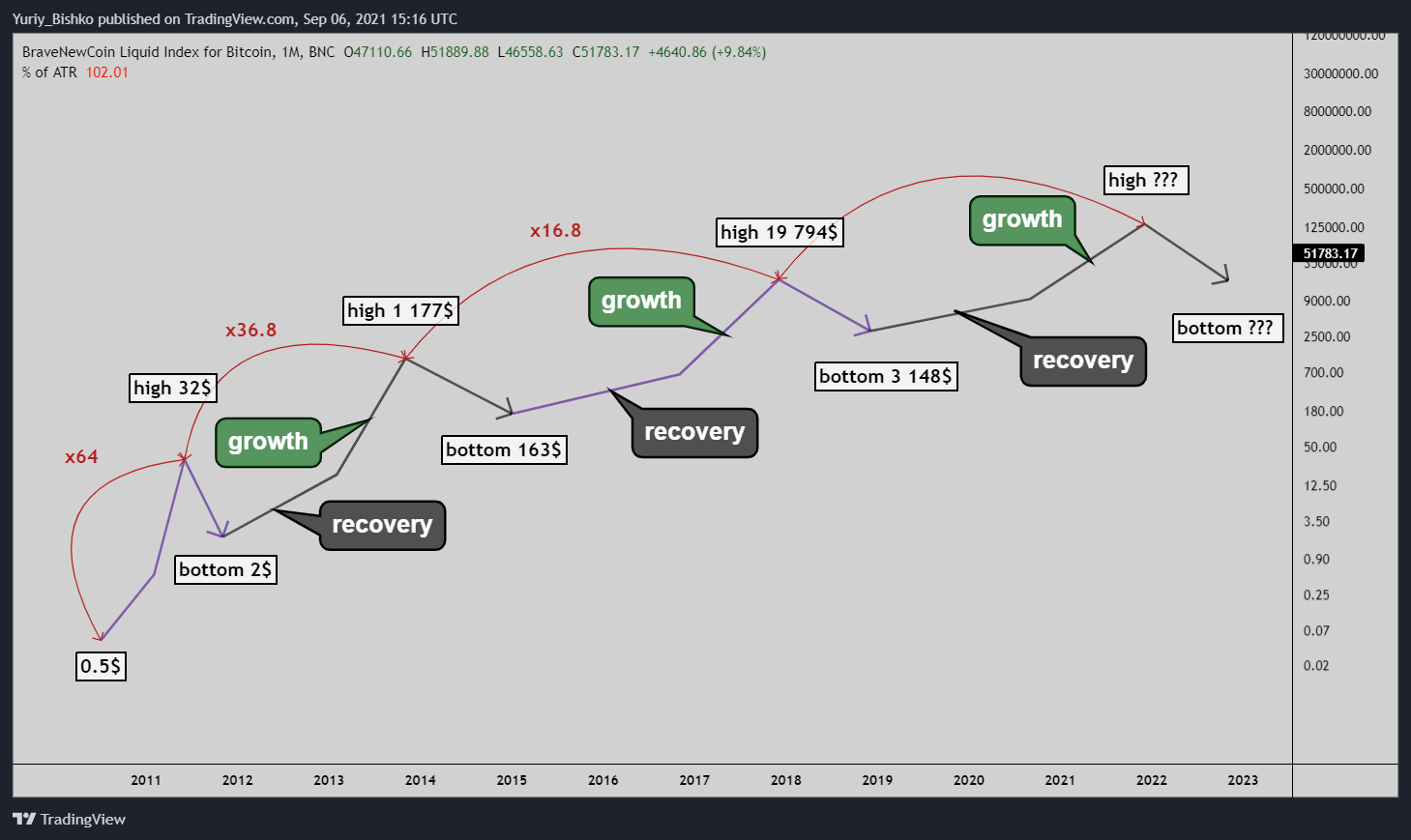If we analyze the growth of bitcoin from the bottom to the maximum of the cycle, we note that with each new cycle, the growth decreased approximately twice. Based on this analysis, we can find the ratio of growth of one cycle to another and approximately find the rate of future growth.

64/36.8 = 1.74 – the ratio between the first two cycles
36.8/16.8 = 2.19 – the ratio between the second and third cycle
(1.74 + 2.19)/2 =1.965 – the average growth rate between cycles
16.8/1.965 = 8.55 – the approximate value of how much bitcoin can grow in this cycle

19 794\$ * 8.55 = 169 238\$ – the approximate value to which bitcoin can grow using this approach.

Based on this hypothesis, the price can reach 169 thousand dollars.

## The second model is logarithmic regression of bitcoin

The presented model makes it possible to make predictions about the future price of bitcoin. In the chart, we see a logarithmic growth channel that narrows relatively to time and the rise in the price of bitcoin. From our point of view, this information should not be used as trade advice, but it should be borne in mind. Each year, this model is adjusted and changed, but despite this, the price responds quite well at the level of the logarithmic channel and can serve as an additional signal to confirm your own trading decisions.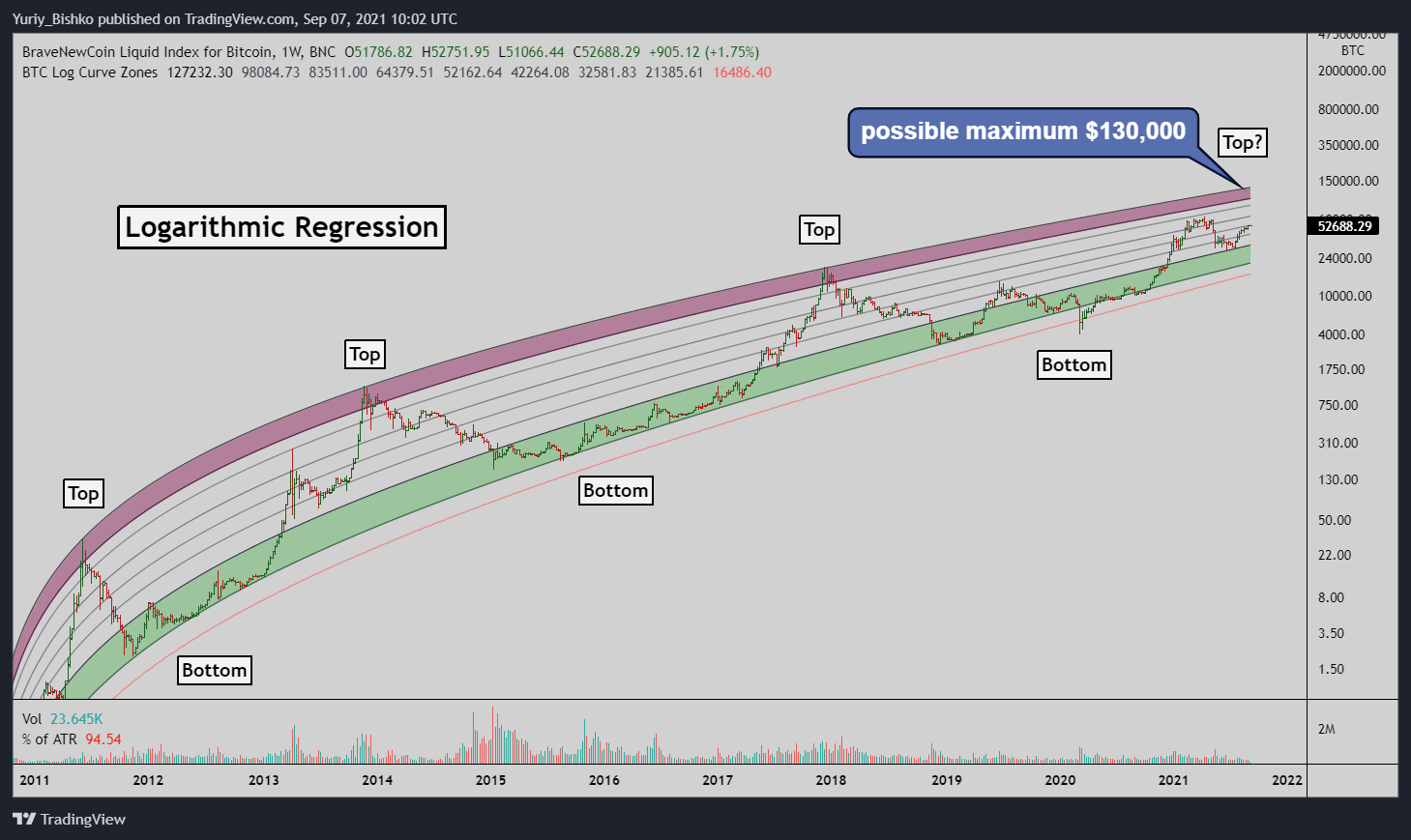Based on the data of this model, the market maximum may be around \$ 130,000. Over time, we will also test this, but this is the third model, including Stock to Flow for BTC, which indicates that the maximum of this bitcoin cycle may be above \$ 100,000.

## The third model is Fibonacci

From the point of view of mathematics, Fibonacci (golden ratio) is an ideal proportion, to which somehow all living and non-living things in nature aspire.

Since man is a part of nature that works by mathematical laws, and the market is a creation of humans, it is not surprising that Fibonacci numbers work in markets. The next model we will consider will be the Fibonacci model.

We highlight the following levels : 0; 1; 1.272; 1.618; 2.272

We place the Fibonacci grid from the maximum to the bottom of the cycle. This way we can predict future marks where the price could potentially come from. On the example of the previous two cycles of Bitcoin, we can see how the price came to the level of 1,618 tested as resistance, then returned and tested the level of 1,272, after which began a rapid rise to the level of 2,272 where the bitcoin cycle ended.

Now history repeats itself, the price has reached 1.618, adjusted to 1,272. In the past, after two or three months, the price reached 2,272. We can’t know for sure whether bitcoin will reach \$ 100,000 to \$ 200,000 by the end of this year, but thanks to these models, we can have a rough global vision of the market that will definitely help our trade.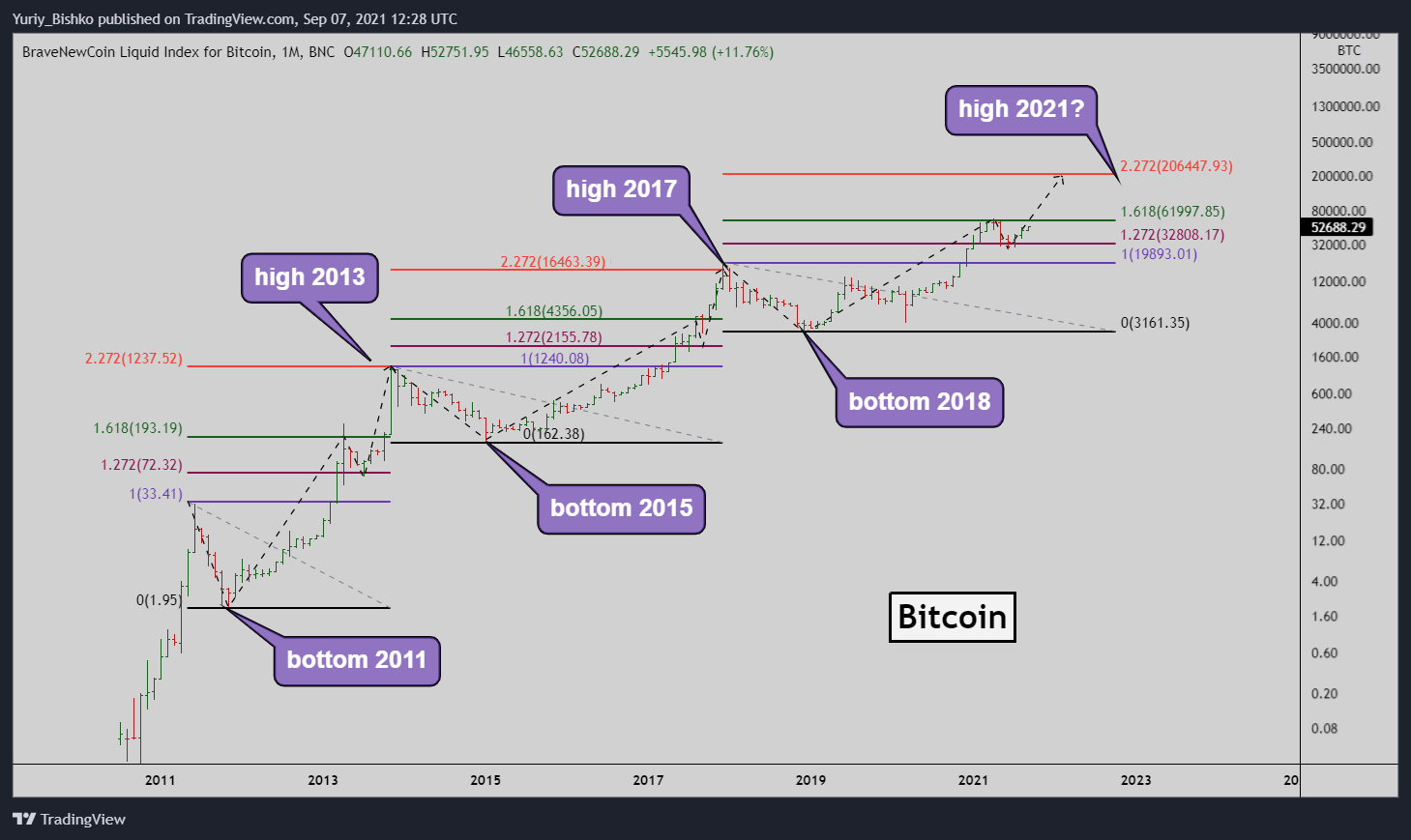## Summary

There are various models that indicate that 65 thousand dollars is not the maximum market. Stock to flow model, and the models presented above suggest that bitcoin may soon cost from \$ 130,000 to \$ 200,000. It is important to understand that this does not mean that all these models will work for sure or it is worth running now and buying bitcoin for all savings. These models can serve as an additional signal that confirms the trading decisions of your strategies.